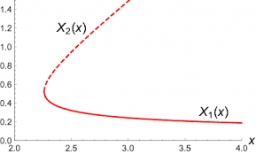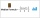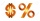# Solve 3

(6n+1) (4n-1) = 3n2

n1 =  0.271
n2 =  -0.1757

### Step-by-step explanation:

Our quadratic equation calculator calculates it.

${n}_{2}=\left(-0.1757\right)=-0.1757$Did you find an error or inaccuracy? Feel free to write us. Thank you!Tips to related online calculators
Looking for help with calculating roots of a quadratic equation?
Do you have a linear equation or system of equations and looking for its solution? Or do you have a quadratic equation?

## Related math problems and questions:

• SequenceWrite the first 6 members of this sequence: a1 = 5 a2 = 7 an+2 = an+1 +2 an
• FruitsAmy bought a basket of fruits 1/5 of them were apples,1/4 were oranges, and the rest were 33 bananas. How many fruits did she buy in all?
• EquationSolve equation and check the result: 1.4x - 3/2 + x - 9,8 = x + 0,4/3 - 7 + 1,6/6Which of the points belong function f:y= 2x2- 3x + 1 : A(-2, 15) B (3,10) C (1,4)
• VCP equationSolve the following equation with variations, combinations and permutations: 4 V(2,x)-3 C(2,x+ 1) - x P(2) = 0
• BoatsThree-quarters of boats are white, 1/7 are blue and 9 are red. How many boats do we have?
• Solve equationsolve equation: [(a2)+3]1/2 + [(a2)-3]1/2 = 5
• Median or middleThe number of hours of television watched per day by a sample of 28 people is given below: 4, 1, 5, 5, 2, 5, 4, 4, 2, 3, 6, 8, 3, 5, 2, 0, 3, 5, 9, 4, 5, 2, 1, 3, 4, 7, 2, 9 What is the median value?
• Simple equationSolve the following simple equation: 2. (4x + 3) = 2-5. (1-x)
• Percent to interval76% is between which of the following two numbers. 1) 1/5 & 1/4. 2) 1/3 & 1/2 3) 2/3 & 7/10 4) 3/4 & 5/6
• Cancel fractionsCompress the expression of factorial: (n+6)!/(n+4)!-n!/(n-2)!
• N percentille problemHere is a data set (n=117) that has been sorted. 10.4 12.2 14.3 15.3 17.1 17.8 18 18.6 19.1 19.9 19.9 20.3 20.6 20.7 20.7 21.2 21.3 22 22.1 22.3 22.8 23 23 23.1 23.5 24.1 24.1 24.4 24.5 24.8 24.9 25.4 25.4 25.5 25.7 25.9 26 26.1 26.2 26.7 26.8 27.5 27.6 2Find the roots of the quadratic equation: 3x2-4x + (-4) = 0.One evening 2/3 of students watch TV. Of those students, 3/8 watched a reality show. Of the students that watched the show, 1/4 of them recorded it. What fraction of the students watched and recorded reality tv.In the arithmetic sequence is given: Sn=2304, d=2, an=95 Calculate a1 and n.Write the equation of the quadratic function, which includes points A (-1, 10), B (2, 19), C (1,4)The division of numbers $a and$b increase by-product of the numbers $c and$d